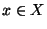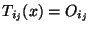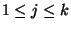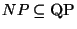Next: NEAREST CODEWORD Up: Miscellaneous Previous: MAXIMUM BETWEENNESS   Index

### MINIMUM DECISION TREE

• INSTANCE: Finite set X of objects, collectionof binary tests.

• SOLUTION: A decision tree t for X using the tests in T, where a decision tree is a binary tree in which each non-leaf vertex is labelled by a test from T, each leaf is labelled by an object from X, the edge from a non-leaf vertex to its left son is labelled 0 and the one to its right son is labelled 1, and, ifis the sequence of vertex and edge labels on the path from the root to a leaf labelled by, then x is the unique object for whichfor all j,.

• MEASURE: Number of leaves of t.

• Comment: Not approximable withinunless.